Updating search results...

# 60 Results

View
Selected filters:
• cl3lesson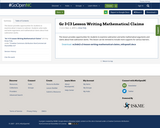Conditional Remix & Share Permitted
CC BY-NC-SA
Rating
0.0 stars

This lesson provides opportunities for students to examine what it means to subtract. Students solve tasks and write arguments and mathematical claims about how subtraction works.

Subject:
Mathematics
Material Type:
Lesson
11/06/2019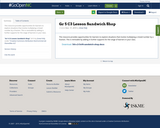Conditional Remix & Share Permitted
CC BY-NC-SA
Rating
0.0 stars

This resource provides opportunities for learners to explore situations that involve multiplying a mixed number by a fraction. This is remixable by adding in further supports for the range of learners in your class.

Subject:
Mathematics
Material Type:
Activity/Lab
Lesson Plan
11/17/2019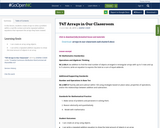Conditional Remix & Share Permitted
CC BY-NC-SA
Rating
0.0 stars

In this lesson, students create arrays to solve a problem within a real-life context and write repeated addition equations that represent the arrays they have created.

Subject:
Mathematics
Material Type:
Lesson
11/06/2019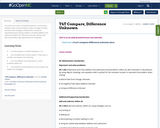Conditional Remix & Share Permitted
CC BY-NC-SA
Rating
0.0 stars

This resource is part of Tools4NCTeachers.

In this lesson, students solve a word problem using a strategy of choice and share the strategy to develop the concept of representing and solving problems involving addition and subtraction within 20. This lesson format may be used with any problem type appropriate for the grade.

Subject:
Mathematics
Material Type:
Lesson
Lesson Plan
11/06/2019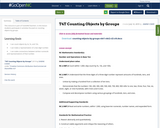Conditional Remix & Share Permitted
CC BY-NC-SA
Rating
0.0 stars

This resource is part of Tools4NCTeachers.

In this lesson students participate in activities focused on counting objects by groups.

Subject:
Mathematics
Material Type:
Lesson
Lesson Plan
11/06/2019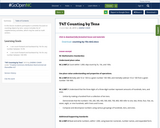Conditional Remix & Share Permitted
CC BY-NC-SA
Rating
0.0 stars

In this lesson students participate in activities focused on counting objects by tens. Lesson includes list of supplementary activities, which may be used as math centers.

Subject:
Mathematics
Material Type:
Lesson
11/06/2019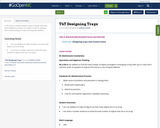Conditional Remix & Share Permitted
CC BY-NC-SA
Rating
0.0 stars

In this lesson, students explore ways to design trays that will fit different amounts of cookies in order to develop their understanding of arrays and repeated addition.

Subject:
Mathematics
Material Type:
Lesson
11/06/2019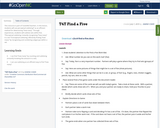Conditional Remix & Share Permitted
CC BY-NC-SA
Rating
0.0 stars

This resource is part of Tools4NCTeachers.

In this lesson, students will use perceptual subitizing* as an efficient method for determining "how many". Through experiences, students will subitize sets within five.

*Perceptual subitizing: instantly recognizing "how many" in a set
*Conceptual subitizing: efficiently finding "how many" by recognizing and combining subgroups within a set.

Subject:
Mathematics
Material Type:
Lesson
Lesson Plan
11/06/2019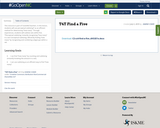Conditional Remix & Share Permitted
CC BY-NC-SA
Rating
0.0 stars

This resource is part of Tools4NCTeachers.

In this lesson, students will use perceptual subitizing* as an efficient method for determining "how many". Through experiences, students will subitize sets within five.

*Perceptual subitizing: instantly recognizing "how many" in a set
Conceptual subitizing: efficiently finding "how many" by recognizing and combining subgroups within a set.

Subject:
Mathematics
Material Type:
Lesson
Lesson Plan
11/06/2019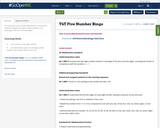Conditional Remix & Share Permitted
CC BY-NC-SA
Rating
0.0 stars

This resource is part of Tools4NCTeachers.

In this lesson, students compare two-digit numbers based on the meanings of the tens and ones digits.

Subject:
Mathematics
Material Type:
Lesson
Lesson Plan
11/06/2019Conditional Remix & Share Permitted
CC BY-NC-SA
Rating
0.0 stars

This lesson is from Tools4NCTeachers. Students explore addition and subtraction Tasks and write mathematical claims about the concepts. This is remixable.

Subject:
Mathematics
Material Type:
Activity/Lab
Formative Assessment
Lesson Plan
11/06/2019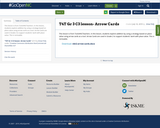Conditional Remix & Share Permitted
CC BY-NC-SA
Rating
0.0 stars

This lesson is from Tools4NCTeachers. In this lesson, students explore addition by using a strategy based on place value using arrow cards as a tool. Arrow Cards are used in Grade 2 to support students' work with place value. This is remixable.

Subject:
Mathematics
Material Type:
Activity/Lab
Lesson Plan
11/06/2019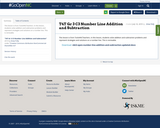Conditional Remix & Share Permitted
CC BY-NC-SA
Rating
0.0 stars

This lesson is from Tools4NCTeachers. In this lesson, students solve addition and subtraction problems and represent strategies and solutions on a number line. This is remixable.

Subject:
Mathematics
Material Type:
Activity/Lab
Formative Assessment
11/06/2019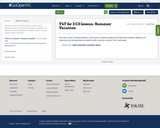Conditional Remix & Share Permitted
CC BY-NC-SA
Rating
0.0 stars

This lesson is from Tools4NCTeachers. In this lesson, students explore the relationship between addition and subtraction by solving distance problems with a vacation context. This is remixable.

Subject:
Mathematics
Material Type:
Activity/Lab
Lesson Plan
11/06/2019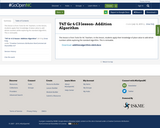Conditional Remix & Share Permitted
CC BY-NC-SA
Rating
0.0 stars

This lesson is from Tools for NC Teachers. In this lesson, students apply their knowledge of place value to add whole numbers while exploring the standard algorithm. This is remixable.

Subject:
Mathematics
Material Type:
Activity/Lab
Lesson Plan
11/06/2019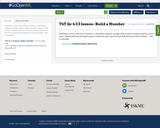Conditional Remix & Share Permitted
CC BY-NC-SA
Rating
0.0 stars

This lesson is from Tools for NC Teachers. In this lesson, students use digit cards to build a number based on a set of clues. Students will have the opportunity to create their own clues and work with partners to build numbers. This is remixable.

Subject:
Mathematics
Material Type:
Activity/Lab
Lesson Plan
11/06/2019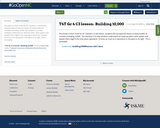Conditional Remix & Share Permitted
CC BY-NC-SA
Rating
0.0 stars

This lesson is from Tools for NC Teachers. In this lesson, students will use base-ten blocks to build models of numbers including 10,000. The intention is to help students understand our base-ten place value system and explain that a digit in the ones place represents 10 times as much as it represents in the place to its right. This is remixable.

Subject:
Mathematics
Material Type:
Activity/Lab
Lesson Plan
11/06/2019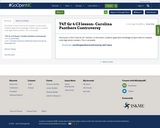Conditional Remix & Share Permitted
CC BY-NC-SA
Rating
0.0 stars

This lesson is from Tools for NC Teachers. In this lesson, students apply their knowledge of place value to compare multi-digit whole numbers. This is remixable.

Subject:
Mathematics
Material Type:
Activity/Lab
Lesson Plan
11/06/2019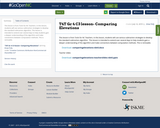Conditional Remix & Share Permitted
CC BY-NC-SA
Rating
0.0 stars

This lesson is from Tools for NC Teachers. In this lesson, students will use various subtraction strategies to develop the standard subtraction algorithm. This lesson is intended to extend over several days to help students gain a deeper understanding of the algorithm and make connections between computation methods. This is remixable.

Subject:
Mathematics
Material Type:
Activity/Lab
Lesson Plan
11/06/2019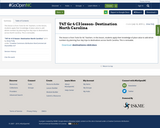Conditional Remix & Share Permitted
CC BY-NC-SA
Rating
0.0 stars

This lesson is from Tools for NC Teachers. In this lesson, students apply their knowledge of place value to add whole numbers by planning four day trips to destinations across North Carolina. This is remixable.

Subject:
Mathematics
Material Type:
Activity/Lab
Lesson Plan